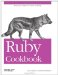# Comparing Two Files

Problem

You want to see if two files contain the same data. If they differ, you might want to represent the differences between them as a string: a patch from one to the other.

Solution

If two files differ, it's likely that their sizes also differ, so you can often solve the problem quickly by comparing sizes. If both files are regular files with the same size, you'll need to look at their contents.

This code does the cheap checks first:

1. If one file exists and the other does not, they're not the same.
2. If neither file exists, say they're the same.
3. If the files are the same file, they're the same.
4. If the files are of different types or sizes, they're not the same.
```	class File
def File.same_contents(p1, p2)
return false if File.exists?(p1) != File.exists?(p2)
return true if !File.exists?(p1)
return true if File.expand_path(p1) == File.expand_path(p2)
return false if File.ftype(p1) != File.ftype(p2) ||
File.size(p1) != File.size(p2)
```

Otherwise, it compares the files contents, a block at a time:

```	 open(p1) do |f1|
open(p2) do |f2|
blocksize = f1.lstat.blksize
same = true
while same && !f1.eof? && !f2.eof?
end
return same
end
end
end
end
```

To illustrate, I'll create two identical files and compare them. I'll then make them slightly different, and compare them again.

```	1.upto(2) do |i|
open("output#{i}", 'w') { |f| f << 'x' * 10000 }
end
File.same_contents('output1', 'output2') # => true
open("output1", 'a') { |f| f << 'x' }
open("output2", 'a') { |f| f << 'y' }
File.same_contents('output1', 'output2') # => false

File.same_contents('nosuchfile', 'output1') # => false
File.same_contents('nosuchfile1', 'nosuchfile2') # => true
```

Discussion

The code in the Solution works well if you only need to determine whether two files are identical. If you need to see the differences between two files, the most useful tool is is Austin Ziegler's Diff::LCS library, available as the diff-lcs gem. It implements a sophisticated diff algorithm that can find the differences between any two enumerable objects, not just strings. You can use its LCS module to represent the differences between two nested arrays, or other complex data structures.

The downside of such flexibility is a poor interface when you just want to diff two files or strings. A diff is represented by an array of Change objects, and though you can traverse this array in helpful ways, there's no simple way to just turn it into a string representation of the sort you might get by running the Unix command diff.

Fortunately, the lcs-diff gem comes with command-line diff programs ldiff and htmldiff. If you need to perform a textual diff from within Ruby code, you can do one of the following:

1. Call out to one of those programs: assuming the gem is installed, this is more portable than relying on the Unix diff command.
2. Import the program's underlying library, and fake a command-line call to it. You'll have to modify your own program's ARGV, at least temporarily.
3. Write Ruby code that copies one of the underlying implementations to do what you want.

Here's some code, adapted from the ldiff command-line program, which builds a string representation of the differences between two strings. The result is something you might see by running ldiff, or the Unix command diff. The most common diff formats are :unified and :context.

```	require 'rubygems'
require 'diff/lcs/hunk'

def diff_as_string(data_old, data_new, format=:unified, context_lines=3)
```

First we massage the data into shape for the diff algorithm:

```	data_old = data_old.split(/
/).map! { |e| e.chomp }
data_new = data_new.split(/
/).map! { |e| e.chomp }
```

Then we perform the diff, and transform each "hunk" of it into a string:

```	 output = ""
diffs =
Diff::LCS.diff(data_old, data_new)
return output if diffs.empty?
oldhunk = hunk = nil
file_length_difference = 0
diffs.each do |piece|
begin
hunk = Diff::LCS::Hunk.new(data_old, data_new, piece, context_lines,
file_length_difference)
file_length_difference = hunk.file_length_difference
next unless oldhunk

# Hunks may overlap, which is why we need to be careful when our
# diff includes lines of context. Otherwise, we might print
# redundant lines.
if (context_lines > 0) and hunk.overlaps?(oldhunk)
hunk.unshift(oldhunk)
else
output << oldhunk.diff(format)
end
ensure
oldhunk = hunk
output << "
"
end
end

#Handle the last remaining hunk
output << oldhunk.diff(format) << "
"
end
```

Here it is in action:

```	s1 = "This is line one.
This is line two.
This is line three.
"
s2 = "This is line 1.
This is line two.
This is line three.
" +
"This is line 4.
"
puts diff_as_string(s1, s2)
# @@ -1,4 +1,5 @@
# -This is line one.
# +This is line 1.
# This is line two.
# This is line three.
# +This is line 4.
```

With all that code, on a Unix system you could be forgiven for just calling out to the Unix diff program:

```	open('old_file', 'w') { |f| f << s1 }
open('new_file', 'w') { |f| f << s2 }

puts %x{diff old_file new_file}
# 1c1
# < This is line one.
# ---
# > This is line 1.
# 3a4
# > This is line 4.
```

• The algorithm-diff gem is another implementation of a general diff algorithm; its API is a little simpler than diff-lcs, but it has the same basic structure; both gems are descended from Perl's Algorithm::Diff module
• It's not available as a gem, but the diff.rb package is a little easier to script from Ruby if you need to create a textual diff of two files; look at how the unixdiff.rb program creates a Diff object and manipulates it (http://users.cybercity.dk/~dsl8950/ruby/diff.html)
• The MD5 checksum is often used in file comparisons: I didn't use it in this recipe because when you're only comparing two files, it's faster to compare their contents; in Recipe 23.7, "Finding Duplicate Files," though, the MD5 checksum is used as a convenient shorthand for the contents of many filesRuby Cookbook (Cookbooks (OReilly))
ISBN: 0596523696
EAN: 2147483647
Year: N/A
Pages: 399